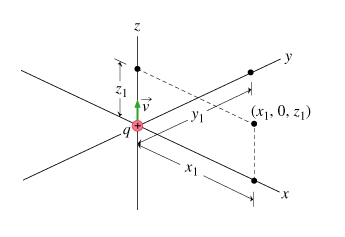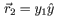# Problem: Magnetic Field near a Moving ChargeA particle with positive charge q is moving with speed v along the z axis toward positive z. At the time of this problem it is located at the origin, x=y=z=0. Your task is to find the magnetic field at various locations in the three-dimensional space around the moving charge. (Figure 1)Find the magnetic field at the point.Express your answer in terms of µ0, q, v, x1, and y1, and use x^, y^, and z^ for the three-unit vectors.

###### FREE Expert Solution

Magnetic field:

94% (454 ratings)###### Problem DetailsMagnetic Field near a Moving Charge

A particle with positive charge q is moving with speed along the z axis toward positive z. At the time of this problem it is located at the origin, x=y=z=0. Your task is to find the magnetic field at various locations in the three-dimensional space around the moving charge. (Figure 1)

Find the magnetic field at the point.

Express your answer in terms of µ0, q, v, x1, and y1, and use x^, y^, and z^ for the three-unit vectors.